2019年05月26日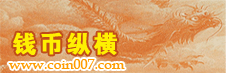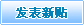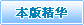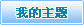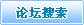本页主题: 与“九”字相关的趣味性流水号码。 人气：250 | 收藏主题 | 上一主题 | 下一主题
scyour [离]#### 与“九”字相关的趣味性流水号码。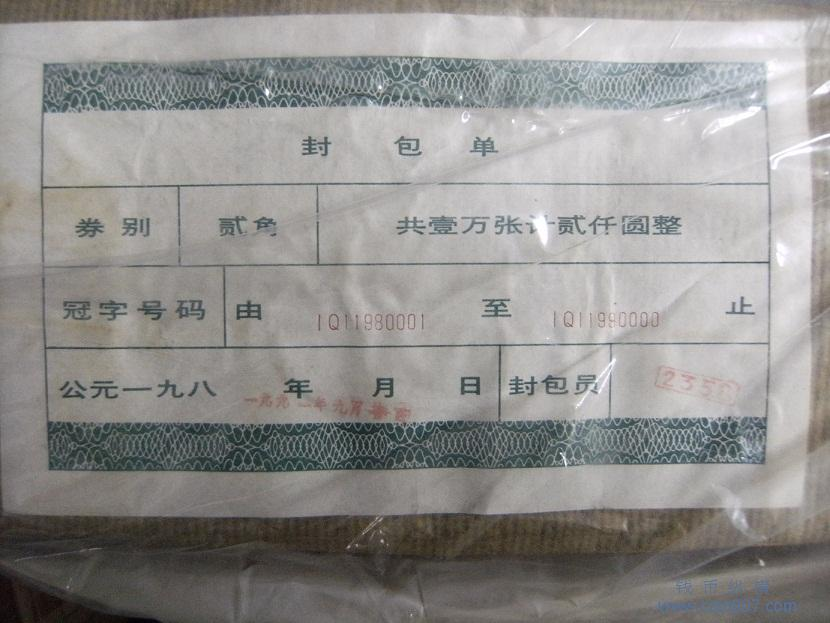512/8=64.  64/8=8.

294912/8=36864.  36864/8=4608.  4608/8=576.  576/8=72.  72/8=9.（除以5个8后等于9）。
scyour [离]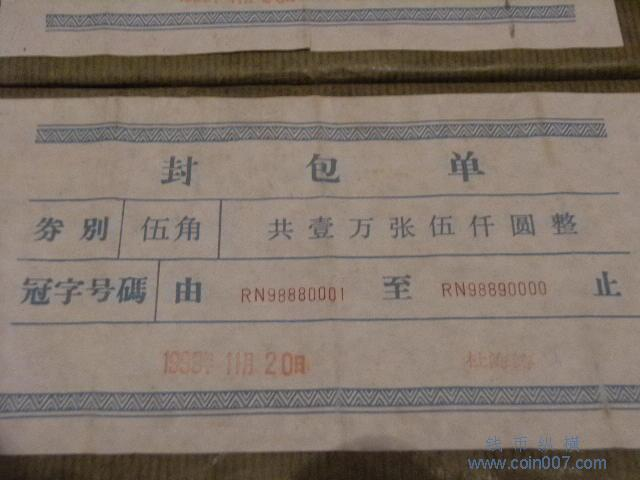32768/8=4096.  4096/8=512.  512/8=64.  64/8=8.

18874368/8=2359296.    2359296/8=294912.  294912/8=36864.  36864/8=4608.

4608/8=576.    576/8=72.  72/8=9.  .（除以7个8后等于9）。
 快速跳至 >> 网站建设  |- 安全支付  |- 建议意见  |- 各类申请  |- 管理团队 >> 专业讨论  |- 独家访谈  |- 历代古钱  |- 机制银币  |- 中国铜元  |- 历代纸钞  |- 银锭研究  |- 铜镜鉴赏  |- 徽章铜章  |- 人民币区  |- 外国货币  |- 邮票磁卡  |- 古玩杂项  |- 花钱栏目 >> 特色论坛  |- 市场行情  |- 微拍预展  |- 代用币区  |- 吉林铜元  |- 川陕货币  |- 评级鉴定  |- 江南银币  |- 四川银币  |- 四川铜元  |- 麦老学堂  |- 东三省毫  |- 机制方孔  |- 大头小头  |- 西域钱币  |- 马兰专区  |- 原创擂台  |- 聚会写真  |- 眼力学习  |- 收藏资料  |- 纠纷调解